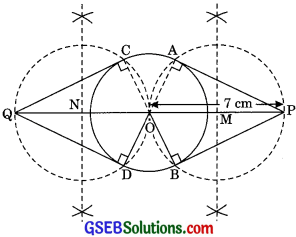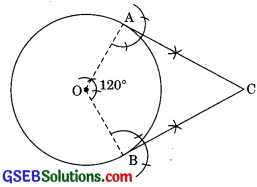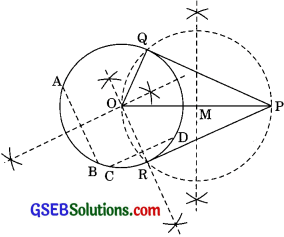# GSEB Solutions Class 10 Maths Chapter 11 Constructions Ex 11.2

Gujarat Board GSEB Solutions Class 10 Maths Chapter 11 Constructions Ex 11.2 Textbook Questions and Answers.

## Gujarat Board Textbook Solutions Class 10 Maths Chapter 11 Constructions Ex 11.2

Question 1.
Draw a circle of radius 6 cm. From a point 10 cm away from its centre, construct the pair of tangents to the circle and measure their lengths.
Solution:
Steps of construction.
1. Draw a circle of radius 6 cm and take O as centre.
2. Take a point P which is 10 cm away from O. Join OP.
3. Bisect OP. Let M be the mid-point of OP.
4. Take M as centre and MO as radius, draw another circle intersecting the previous circle at Q and R.
5. Join PQ and PR
PQ and PR are the required tangents
PQ = PR = 8cm.

Justification:
Join OQ and OR.∠OQP = ∠ORP = 90° [Angles in semicircies]
Since OQ and OR are radii of the circle and PQ and PR will be the tangents to the circle at Q and R respectively. Circle with diameter OP intersects the given circle in only two points.
Hence, only two tangents can be drawn.

Question 2.
Construct a tangent to a circle of radius 4 cm from a point on the concentric circle of radius 6 cm and measure its length. Also verify the measurement by actual calculation.
Solution:
Steps of Construction:
1. Draw a circle of radius 4 cm with centre O.
2. Taking O as centre draw another circle of radius 6 cm.
3. Take a point P an outer circle. Join OP.
4. Bisect OP. Let M be the mid-point of OP.
5. Take M as centre and MO as radius, draw a circle. Let it intersect the given circle at the point Q and R.
6. Join PQ.
PQ is the required tangent. PQ = 4.5 cm.
By actual Calculation
As ∠OQP = 90° (Angle in a semicircle)By Pythagoras theorem:
OP2 = PQ2 + OQ2
PQ = $$\sqrt{\mathrm{OP}^{2}-\mathrm{OQ}^{2}}$$
= $$\sqrt{6^{2}-4^{2}}$$
= $$\sqrt{36-16}$$ = $$\sqrt{20}$$
= 4.47 cm (approx)

Justification:
Since ∠OQP = 90° (Angle in a semicircle)
⇒ PQ ⊥ OQ
Since OQ is the radius of the given circle, PQ has to be a tangent to the circle.Question 3.
Draw a circle of radius 3 cm. Take two points P and Q on one of its extended diameter each at a distance of 7 cm from its centre. Draw tangents to the circle from these two points P and Q.
Solution:
Steps of construction:
1. Draw a circle of radius 3 cm of centre O.
2. Take two points P arid Q on one of its extended diameter each at a distance 7 cm from its centre O.
3. Bisect PO. Let M be the mid-point of PO.
4. Take M as centre and MO as radius, draw a circle intersecting the given circle at A and B5. Join PA and PB.
6. Bisect QO. Let N be the mid-point of QO.
7. Take N as centre and NO as radius, draw a circle intersecting the given circle of C and D.
8. Join QC and QD.
PA, PB, QC and QD are the required tangents.

Justification:
Join OA and OB.
⇒ ∠PAO = 90° [Angle in Semicircle]
PA ⊥ OA
Since OA is the radius of the given circle PA has to be a tangent to the circle. Similarly, PB in also tangent to the circle. ,.
With the same above explanation, QC and QD are also tangent to the circle.Question 4.
Draw a pair of tangents to a circle to radius 5 cm which are inclined to each other at an angle of 60°.
Solution:
Steps of construction
1. Draw a circle of radius 5 cm and take O as centre.
2. Take a point A on a circle and draw ∠AOB = 120°.
3. At A and B draw angles of 90° which other arms meet at C. Then, AC and BC are the required tangents inclining each other at an angle of 60°.Justification:
∠OAC = 90° [By construction]
∠OBC = 90°
⇒ OA ⊥ AC
OB ⊥ BC
Also, OA and OB are the radii of the circle.
∴ AC and BC is a tangent to the circle. In quadrilateral ΔOBC,
∠AOB + ∠OBC + ∠BCA + ∠CAO = 360°
[Angle sum property of a quadrilaterall
⇒ 120° + 90° + ∠BCA + 90° = 360°
⇒ ∠BCA + 300° = 360°
⇒ ∠BCA = 360° – 300° = 60°Question 5.
Draw a line segment AB of length 8 cm. Taking A as the centre, draw a circle of radius 4 cm and taking B as centre, draw another circle of radius 3 cm. Construct tangents to each circle from the centre of the other circle.
Solution:
Steps of Construction:
1. Draw a line segment AB = 8 cm.
2. Taking A as the centre, draw a circle of radius 4 cm
3. Now taking B as centre, draw a circle of radius 3 cm.
4. Bisect AB. Let M be the mid-point of AB.
5. Taking M as a centre and AM as radius, draw a circle, intersecting the circle with centre A at P and Q; and intersecting the circle with centre B at R and S.6. Join BP and BQ
7. Join AR and AS BP, BQ, AR and AS are the required tangents.

Justification:
∠APB = 90° [Angles in the semicircle]
∠ARB = 90°
BP ⊥ AP
and AR ⊥BR
Since AP and BR are the radii of the circles with A and B as centre respectively. So, BP and AR are the tangents to the circle with centre A and B respectively.Question 6.
Let ABC be a right triangle in which AB = 6cm, BC = 8cm and ∠B = 90°. BD is perpendicular from B on AC. The circle through B, C, D is drawn. Construct the tangents from A to this circle.
Solution:
Steps of Construction:
1. Draw a ABC with AB 6 cm, BC = 8 cm and ∠B = 90°.
2. Draw BD ⊥ AC.
3. Through points B, C and D, draw a circle and mark the centre as O.
4. Join AO and bisect it. Let M be the midpoint of AO.
5. Taking M as centre and MO as radius, draw a circle intersecting the given circle at B and E.6. Join AB and AE.
Thus, AB and AE are the required tangents.

Justification:
By joining OE.
∠AEO = 90° [Angle in the semicircie]
⇒ AE ⊥ OE
Now since OE is a radius of the given circle, AE will be a tangent to the circle.Question 7.
Draw a circle with the help of a bangle. Take a point outside the circle. Construction the pair of tangents from this point to the circle.Steps of Construction:
1. Draw a circle with the help of a bangle.
2. Take two chords AB and CD (non-parallel to each other)
3. Draw the perpendicular bisectors of AB and CD intersecting at O. Then O is the centre of the given circle.
4. Take a point P outside the circle. Join OP
5. Bisect OP. Let M be the mid-point of OP.
6. Taking M as centre and MO as radius draw a circle intersecting the given circle at Q and R.
7. Join PQ and PR
∴ PQ and PR are the required tangents.

Justification:
Join OQ and OR
∠PQO = 90° [Angle in the semicircle]
⇒ PQ ⊥ OQ
Since OQ in a radius of the given circle. PQ will be a tangent to the circle. Similarly, PR will also be a tangent to the circle.